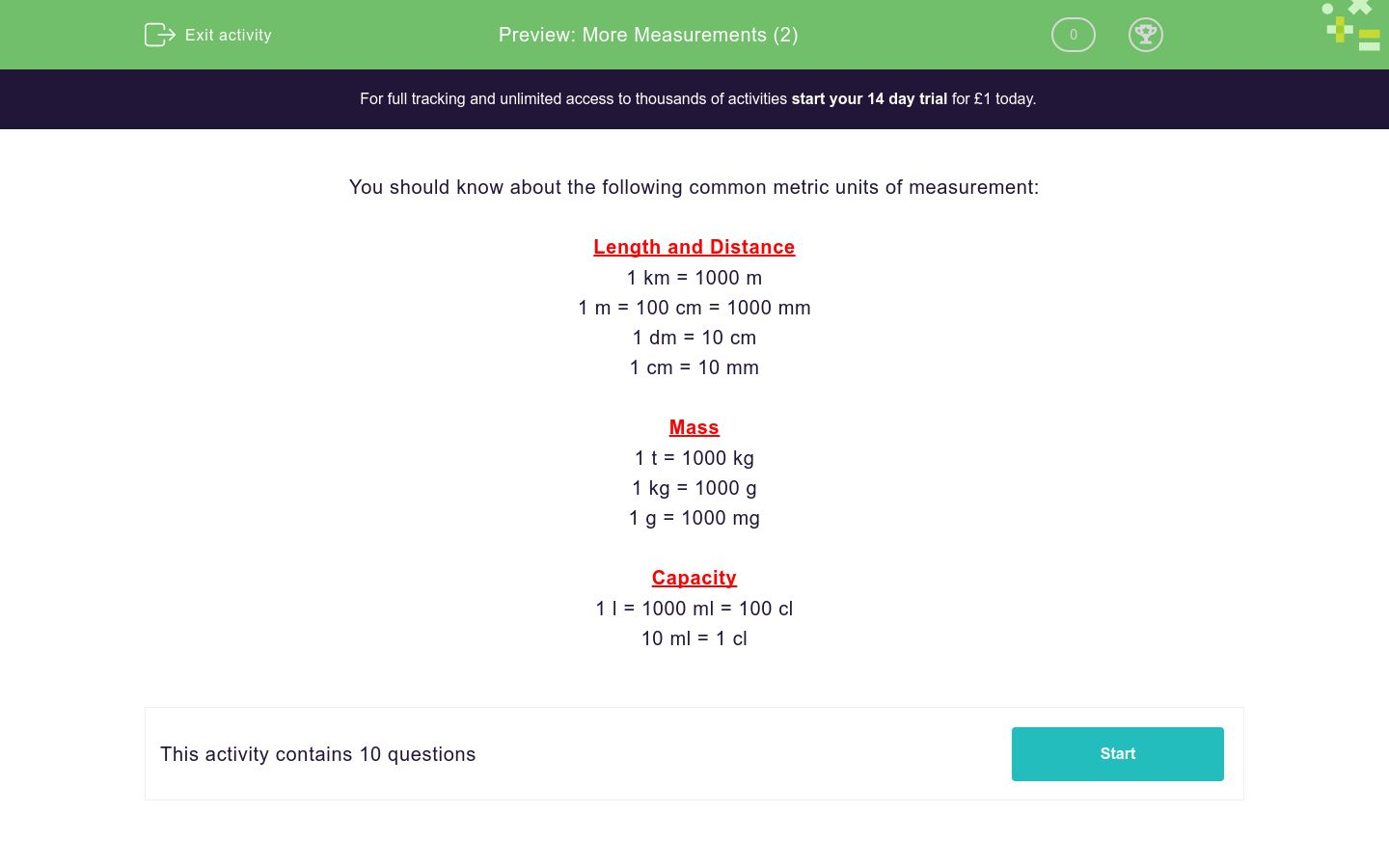# More Measurements (2)

In this worksheet, students compare metric measurements given in different units and state which is more.Key stage:  KS 3

Curriculum topic:   Number

Curriculum subtopic:   Use Standard Units of Measure

Difficulty level:### QUESTION 1 of 10

You should know about the following common metric units of measurement:

Length and Distance

1 km = 1000 m

1 m = 100 cm = 1000 mm

1 dm = 10 cm

1 cm = 10 mm

Mass

1 t = 1000 kg

1 kg = 1000 g

1 g = 1000 mg

Capacity

1 l = 1000 ml = 100 cl

10 ml = 1 cl

Which is more?

1520 ml

1.5 l

they are equal

Which is more?

205 cl

2 l

they are equal

Which is more?

2.5 dm

30 cm

they are equal

Which is more?

185 mm

18.4 cm

they are equal

Which is more?

1950 mm

20 dm

they are equal

Which is more?

1950 kg

2.5 t

they are equal

Which is more?

5150 g

5.1 kg

they are equal

Which is more?

5.2 mm

2.5 cm

they are equal

Which is more?

195 mg

2 g

they are equal

Which is more?

2750000 mg

2.8 kg

they are equal

• Question 1

Which is more?

1520 ml
• Question 2

Which is more?

205 cl
• Question 3

Which is more?

30 cm
• Question 4

Which is more?

185 mm
• Question 5

Which is more?

20 dm
• Question 6

Which is more?

2.5 t
• Question 7

Which is more?

5150 g
• Question 8

Which is more?

2.5 cm
• Question 9

Which is more?

2 g
• Question 10

Which is more?